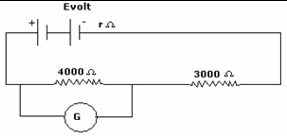# Topic: Current Electricity (Test 1)

Topic: Current Electricity
Q.1
In the measurement of resistance by a metre bridge, the current is necessarily reversed through the bridge wire to eliminate
A. Index errors
B. Random errors
C. Error due to thermoelectric effects
D. End errors
Explaination / Solution:

The connecting metal strips include a small resistance in the circuit called the end resistance. On reversing the current, the end resistance tends to get cancelled out.

Workspace
Report
Q.2
If 2% of the main current is to be passed through the galvanometer of resistance G, the resistance of shunt required is
A. G/50
B. 49G
C. G/49
D. 50G
Explaination / Solution:

The current Ithrough a galvanometer of resistance G is 0.02 I where I is the main current.

The shunt resistance S is calculated as follows.Workspace
Report
Q.3
In the figure, when an ideal voltmeter is connected across 4000 ohm resistance, it reads 30 volts. If the voltmeter is connected across 3000 ohms resistance. It will readA. 35 volt
B. 22.5 volt
C. 20 volt
D. 40 volt
Explaination / Solution:

An ideal voltmeter does not draw any current. Therefore the resistances 4000 Ω and 3000 Ω are in series. The current in the circuit

The potential difference across the 3000 Ω resistorWorkspace
Report
Q.4
According to Ohm's law
A. The electric current I flowing through a substance is independent of the voltage V across its ends
B. The electric current I flowing through a substance is proportional to the square of voltage V across its ends
C. The electric current I flowing through a substance is proportional to the voltage V across its ends
D. The electric current I flowing through a substance is inversely proportional to the voltage V across its ends
Explaination / Solution:

Ohm’s law states I is proportional to V. This holds good at steady temperatures and for the flow of constant current.

Workspace
Report
Q.5
Drift is the random motion of the charged particles within a conductor,
A. along with a very slow net motion in the opposite direction of the field
B. along with accelerated motion in the direction of the field
C. along with zero motion in the direction of the field
D. along with a decelerated motion in the direction of the field
Explaination / Solution:

The electrons in a conductor have random velocities and when an electric field is applied, they suffer repeated collisions and in the process move with a small average velocity, opposite to the direction of the field. This is equivalent to positive charge flowing in the direction of the field.

Workspace
Report
Q.6
orders of magnitude of random electron motion speed to drift speed are like
A. 106m/s,104m/s
B. 102m/s,102m/s
C. 104m/s,102m/s
D. 103m/s,101m/s
Explaination / Solution:

The random velocities of electrons is of the order 105 to 106 m/s, while the drift velocities are of the order 0.1mm/s (10-4m/s)

Workspace
Report
Q.7
The resistance of a metallic conductor increases due to
A. Change in carrier density
B. increase in the number of collisions between the carriers
C. Change in dimensions of the conductor
D. increase in the rate of collisions between the carriers and vibrating atoms of the conductor
Explaination / Solution:

Resistance of a metal of length l , area A is  where n is the carrier density, m the mass of the electron , e the charge of the electron and , the average time between two collisions. at constant temperature, n and  remain constant.  is constant at constant temperature and is called $\rho$ The resistance increases if the length increases or the area decreases.

Workspace
Report
Q.8
A piece of copper and another of germanium are cooled from room temperature to 80K. The resistance
A. of each of them decreases
B. copper decreases and that of germanium increases
C. copper increases and that of germanium decreases
D. of each of them increases
Explaination / Solution:

Copper is a conductor and we know that for conductors, resistance is directly proprtional to temperature. Therefore on decreasing temperature resistance also decreases. Whereas, germanium is a semiconductor and for semiconductors, resistance is inversely proportional to temperature. So on decreasing temperature resistance increases.

Workspace
Report
Q.9
The equivalent resistance of two resistances P and Q which are in series is
A. P×P/(P+Q)
B. Q×Q/(P + Q)
C. P + Q
D. PQ/(P + Q)
Explaination / Solution:

Equivalent resistance of two resistances connected in series is equal to the sum of the two resistances.

Workspace
Report
Q.10
Two cells of 1.25 V and 0.75 V are connected in series with anode of one connected to cathode of the other . The effective voltage will be
A. 0.50 V
B. 2.0 V
C. 1.25 V
D. 0.75 V